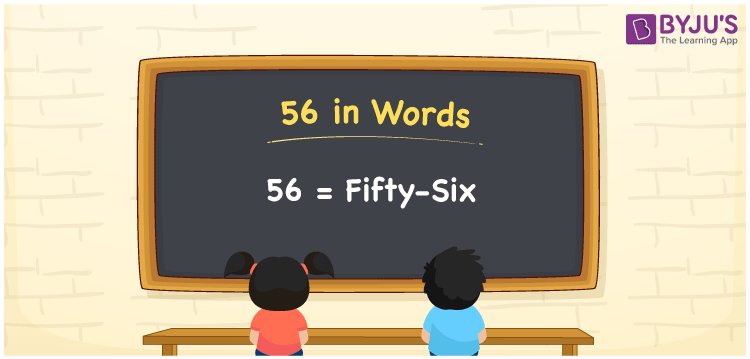# 56 in Words

56 in words is written as Fifty-six. In both the International System of Numerals and the Indian System of Numerals, 56 is written as Fifty-six. The number 56 is a Cardinal Number as it represents some quantity. For example, “there are 56 students present in the class”. Here, 56 quantifies the number of students.

 56 in words Fifty-six Fifty-six in Number 56

## 56 in English Words

56 in English words is read as “Fifty-six”## How to Write 56 in Words?

To write 56 in words, we shall use the place value chart. In the place value chart, put 5 in the tens place and 6 in the ones place. Let us make a place value chart to write the number 56 in words.

 Tens Ones 5 6

Thus, we can write the expanded form as

5 × Ten + 6 × One

= 5 × 10 + 6 × 1

= 50 + 6

= 56

= Fifty-six.

56 is a natural number between 55 and 57.

56 in words – Fifty-six

• Is 56 an odd number? – No
• Is 56 an even number? – Yes
• Is 56 a perfect square number? – No
• Is 56 a perfect cube number? – No
• Is 56 a prime number? – No
• Is 56 a composite number? – Yes

## Frequently Asked Questions on 56 in Words

Q1

### How to write 56 in words?

56 in words is written as Fifty-six.
Q2

### How to write 56 in words in the International and Indian System of Numerals?

In both, the system of numerals, 56 in words, is written as Fifty-six.
Q3

### How to write 56 in a place value chart?

In the place value chart, write 5 in the tens place and 6 in the ones place.